# 四、数据类型

``````type(3)
int
type(3/1)
float
``````

``````x = 3
type(x) # The type of x is an int, not a name
int
``````

``````type(abs)
builtin_function_or_method
``````

## 字符串

``````"data" + "science"
'datascience'
``````

``````"data" + " " + "science"
'data science'
``````

``````"This won't work with a single-quoted string!"
"This won't work with a single-quoted string!"
``````

`str`函数返回任何值的字符串表示形式。 使用此函数，可以构建具有嵌入值的字符串。

``````"That's " + str(1 + 1) + ' ' + str(True)
"That's 2 True"
``````

## 字符串方法

``````"loud".upper()
'LOUD'
``````

``````'hitchhiker'.replace('hi', 'ma')
'matchmaker'
``````

``````s = "train"
t = s.replace('t', 'ing')
u = t.replace('in', 'de')
u
``````

``````s
'train'
``````

## 比较

``````3 > 1 + 1
True
``````

`True`表明这个比较是有效的；Python 已经证实了`3``1 + 1`之间关系的这个简单的事实。下面列出了一整套通用的比较运算符。

``````1 < 1 + 1 < 3
True
``````

``````x = 12
y = 5
min(x, y) <= (x+y)/2 <= max(x, y)
True
``````

``````"Dog" > "Catastrophe" > "Cat"
True
``````

## 序列

``````sum(highs)/len(highs)
14.434000000000001
``````

### 日高温均值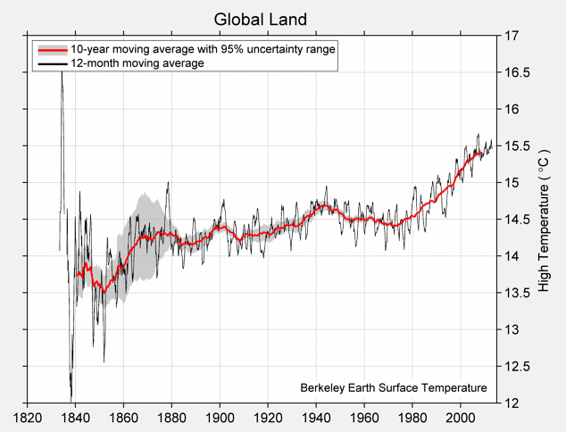### 日低温均值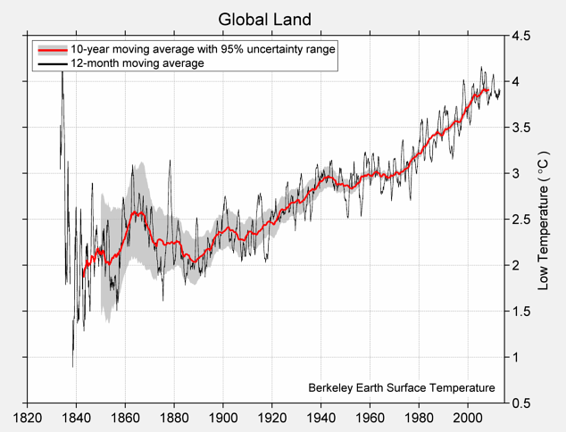## 数组

Python 中有很多种类的集合，我们在这门课中主要使用数组。 我们已经看到，`make_array`函数可以用来创建数值的数组。

``````english_parts_of_speech = make_array("noun", "pronoun", "verb", "adverb", "adjective", "conjunction", "preposition", "interjection")
english_parts_of_speech
'preposition', 'interjection'],
dtype='<U12')
``````

``````import numpy as np
make_array = lambda *args: np.asarray(args)
``````

``````baseline_high = 14.48
highs = make_array(baseline_high - 0.880,
baseline_high - 0.093,
baseline_high + 0.105,
baseline_high + 0.684)
highs
array([ 13.6  ,  14.387,  14.585,  15.164])
``````

``````(9/5) * highs + 32
array([ 56.48  ,  57.8966,  58.253 ,  59.2952])
``````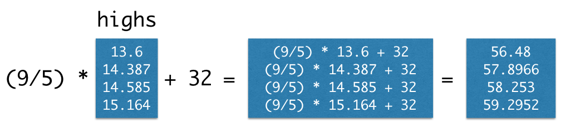``````highs.size
4
highs.sum()
57.736000000000004
highs.mean()
14.434000000000001
``````

### 数组上的函数

`numpy`包，在程序中缩写为`np`，为 Python 程序员提供了创建和操作数组的，方便而强大的函数。

``````import numpy as np
``````

``````np.diff(highs)
array([ 0.787,  0.198,  0.579])
``````

`np.prod` 将所有元素相乘
`np.sum` 将所有元素相加
`np.all` 测试是否所有元素是真值 （非零数值是真值）
`np.any` 测试是否任意元素是真值（非零数值是真值）
`np.count_nonzero` 计算非零元素的数量

`np.char.lower` 将每个元素变成小写
`np.char.upper` 将每个元素变成大写
`np.char.strip` 移除每个元素开头或末尾的空格
`np.char.isalpha` 每个元素是否只含有字母（没有数字或者符号）
`np.char.isnumeric` 每个元素是否只含有数字（没有字母）

np.char.count 在数组的元素中，计算搜索字符串的出现次数
np.char.find 在每个元素中，搜索字符串的首次出现位置
np.char.rfind 在每个元素中，搜索字符串的最后一次出现位置
np.char.startswith 每个字符串是否以搜索字符串起始

## 范围

``````np.arange(end): An array starting with 0 of increasing consecutive integers, stopping before end.
np.arange(5)
array([0, 1, 2, 3, 4])
``````

``````np.arange(start, end): An array of consecutive increasing integers from start, stopping before end.
np.arange(3, 9)
array([3, 4, 5, 6, 7, 8])
np.arange(start, end, step): A range with a difference of step between each pair of consecutive values, starting from start and stopping before end.
np.arange(3, 30, 5)
array([ 3,  8, 13, 18, 23, 28])
``````

``````np.arange(1.5, -2, -0.5)
array([ 1.5,  1. ,  0.5,  0. , -0.5, -1. , -1.5])
``````

### 示例：莱布尼茨的 π 公式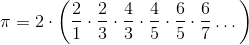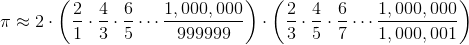令人惊讶的是，当我们将无限多个分数相加时，顺序可能很重要。但是我们对 π 的近似只使用了大量的数量有限的分数，所以可以按照任何方便的顺序，将这些项相加。

``````by_four_to_20 = np.arange(1, 20, 4)
by_four_to_20
array([ 1,  5,  9, 13, 17])
``````

``````positive_term_denominators = np.arange(1, 10000, 4)
positive_term_denominators
array([   1,    5,    9, ..., 9989, 9993, 9997])
``````

``````positive_terms = 1 / positive_term_denominators
``````

``````negative_terms = 1 / (positive_term_denominators + 2)
``````

``````4 * ( sum(positive_terms) - sum(negative_terms) )
3.1413926535917955
``````